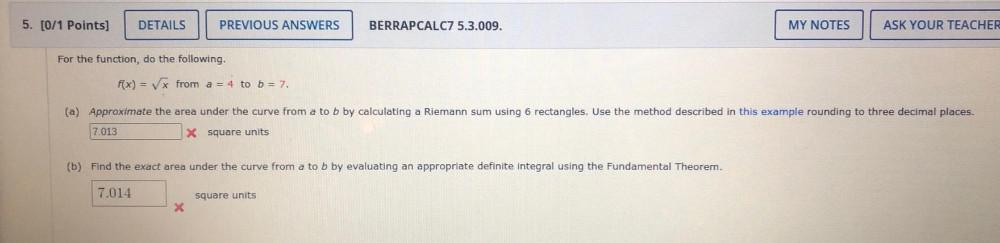Question:

# 5. [0/1 Points] DETAILS PREVIOUS ANSWERS BERRAPCALC7 5.3.009. MY NOTES ASK YOUR TEACHER For the function, do the following. f(x)5. [0/1 Points] DETAILS PREVIOUS ANSWERS BERRAPCALC7 5.3.009. MY NOTES ASK YOUR TEACHER For the function, do the following. f(x) = from a = 4 to b = 7. (a) Approximate the area under the curve from a to b by calculating a Riemann sum using 6 rectangles. Use the method described in this example rounding to three decimal places. 7.013 * Square units (b) Find the exact area under the curve from a to b by evaluating an appropriate definite integral using the Fundamental Theorem. 7.014 square units